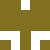#@alendr

## Exercise #1.5.4 -

updated September 15, 2021 ·C
```#include <stdio.h>
#define IN 1
#define OUT 0
/* Count lines, words and characters in Input */

int main() {
int c, nl, nw, nc, state;
state = OUT;
nl = nw = nc = 0;
while ((c = getchar()) != EOF) {
++nc; ```

## Exercise #1.10 - Copy Input to Output

updated September 15, 2021 ·C
```#include <stdio.h>
/* replace tabs, backslashes and
backspaces - with visible characters */
int main() {
int c;

while ((c = getchar()) != EOF) {
if (c == '\t')
printf("\\t");
if (c == '\b')
printf("\\b"); ```

## Exercise #1.10 - Copy Input to Output

updated September 15, 2021 ·C
```#include <stdio.h>
/* replace tabs, backslashes and
backspaces - with visible characters */
int main() {
int c;

while ((c = getchar()) != EOF) {
if (c == '\t')
printf("\\t");
if (c == '\b')
printf("\\b"); ```

## Exercise 1.10:

September 15, 2021 ·NodeJS
```#include <stdio.h>
/* replace tabs, backslashes and
backspaces - with visible characters */
int main() {
int c;

while ((c = getchar()) != EOF) {
if (c == '\t')
printf("\\t");
if (c == '\b')
printf("\\b"); ```

## Exercise 1.9:

updated September 15, 2021 ·C
```#include <stdio.h>
#define NONBLANK 'a'
/* replace string of blanks with single blank */

int main() {
int c, lastc;
lastc = NONBLANK;
while ((c = getchar()) != EOF) {
if (c != ' ')
putchar(c); ```

## Exercise #1.8

updated September 15, 2021 ·C
```#include <stdio.h>
/* Counts blanks, tabs, newlines. */
int main() {
int c, nnl, nb, nt;
nnl = 0; /* number of newlines */
nb = 0; /* number of blanks */
nt = 0; /* number of tabs */
while ((c = getchar()) != EOF)
{if (c == ' ')
++nb;
if (c == '\t') ```

## Exercise #1.5.3 - Line Counting

updated September 15, 2021 ·C
```#include <stdio.h>
/* Count lines in input */
int main() {
int c, nl;
nl = 0;
while ((c = getchar()) != EOF)
if (c == '\n')
++nl;
printf("%d\n", nl);
return 0;
}```

## Exercise #1.5.2 - Character Counting - 2nd Ver

updated September 15, 2021 ·C
```#include <stdio.h>/* Count characters as input */
int main() {
double nc;
for (nc = 0; getchar() != EOF; ++nc)
;

printf("%.0f\n", nc);
return 0;
}```

## Exercise #1.5.2 - Character Counting

updated September 15, 2021 ·C
```#include <stdio.h>
/* Count characters in input. */
int main() {
long nc;
while (getchar() != EOF)
++nc;
printf("%ld\n", nc);
return 0;
}```

## Exercise #1-7

updated September 15, 2021 ·C
```#include <stdio.h>
/* Prg prints EOF */
int main() {
printf("EOF is %d\n", EOF);

}```

## Exercise #1-6

updated September 15, 2021 ·C
```#include <stdio.h>
/* While getchar has a character - 1 is assigned to 'c'.
As soon as EOF is encountered - 0 is assigned &a While loop ends. */
int main()
{
int c;
while (c = getchar() != EOF)
printf("%d\n", c);

printf("%d - at EOF\n", c);
```

## Exercise# 1.5.2 - Character Count

updated September 15, 2021 ·C
```#include <stdio.h>
/* count characters in input; 1st version */

int main() {
long nc;
nc = 0;
while (getchar() != EOF)
++nc;
printf("%1d\n", nc);
}```

## Exercise #1.6 - File Copying. Verify

updated September 14, 2021 ·C
```#include <stdio.h>
/* Copy input to output: - 2nd ver */

int main() {
int c;
while ((c = getchar()) != EOF)
putchar(c);
if ((c = getchar()) != EOF) = 0
printf("It is 0")
else
printf("It is 1"); ```

## Exercise #1.5 - File Copy

updated September 14, 2021 ·C
```#include <stdio.h>
/* Copy input to output: - 2nd ver */

int main() {
int c;
while ((c = getchar()) != EOF)
putchar(c);
printf("\nInteger =    %d\n", EOF);
printf("%d", EOF);
} ```

## Exercise #1.4 - Symbolic Constants

updated September 14, 2021 ·C
```#include <stdio.h>
/* print Fahrenheit-Celcius table
for fahr = 0, 20, ...., 300 */
/* Using Symbolic constants: #define / name / replacement text */

int main()
{
/* int fahr, celsius; */
#define LOWER 0
#define UPPER 300
#define STEP 20 ```

## Exercise 1.2 - Fahrenheit-Celsius Table

updated September 14, 2021 ·C
```#include <stdio.h>
/* print Fahrenheit-Celcius table
for fahr = 0, 20, ...., 300 */

int main()
{
/* int fahr, celsius; */
float fahr, celsius;
int lower, upper, step;

lower = 0; ```

## HelloWorld

September 14, 2021 ·C
```#include <stdio.h>

int main() {
printf("Hello world!\n");
return 0;
}```